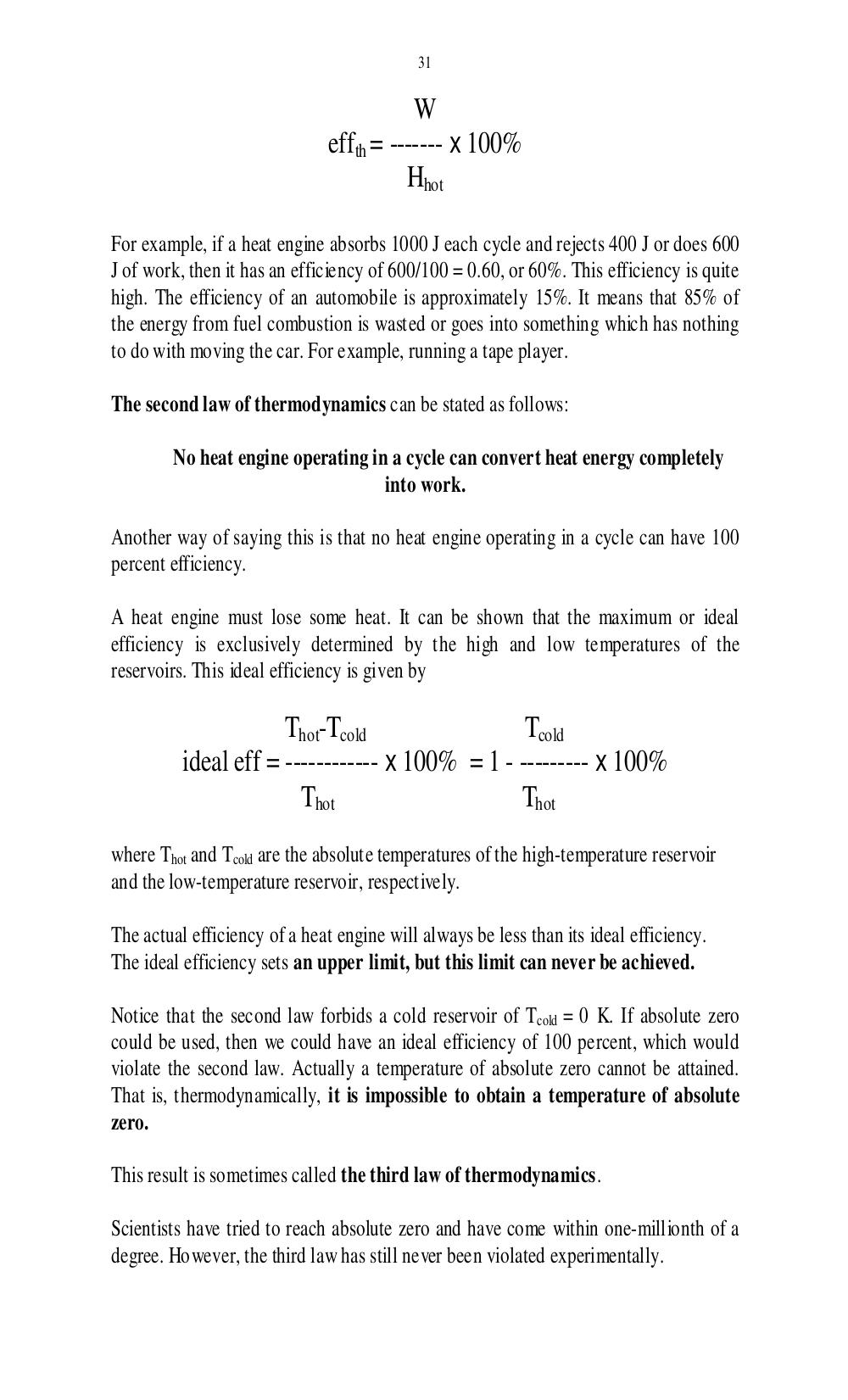# Учебно-методическое пособие по чтению специальной литературы для студентов 1 курса физического факультета. Дроздова И.В - 31 стр.

Составители:

Рубрика:

• ## Иностранный язык

31
W
eff
th
= ------- x 100%
H
hot
For example, if a heat engine absorbs 1000 J each cycle and rejects 400 J or does 600
J of work, then it has an efficiency of 600/100 = 0.60, or 60%. This efficiency is quite
high. The efficiency of an automobile is approximately 15%. It means that 85% of
the energy from fuel combustion is wasted or goes into something which has nothing
to do with moving the car. For example, running a tape player.
The second law of thermodynamics can be stated as follows:
No heat engine operating in a cycle can convert heat energy completely
into work.
Another way of saying this is that no heat engine operating in a cycle can have 100
percent efficiency.
A heat engine must lose some heat. It can be shown that the maximum or ideal
efficiency is exclusively determined by the high and low temperatures of the
reservoirs. This ideal efficiency is given by
T
hot
-T
cold
T
cold
ideal eff = ------------ x 100% = 1 - --------- x 100%
T
hot
T
hot
where T
hot
and T
cold
are the absolute temperatures of the high-temperature reservoir
and the low-temperature reservoir, respectively.
The actual efficiency of a heat engine will always be less than its ideal efficiency.
The ideal efficiency sets an upper limit, but this limit can never be achieved.
Notice that the second law forbids a cold reservoir of T
cold
= 0 K. If absolute zero
could be used, then we could have an ideal efficiency of 100 percent, which would
violate the second law. Actually a temperature of absolute zero cannot be attained.
That is, thermodynamically, it is impossible to obtain a temperature of absolute
zero.
This result is sometimes called the third law of thermodynamics.
Scientists have tried to reach absolute zero and have come within one-millionth of a
degree. However, the third law has still never been violated experimentally.31

W
effth = ------- x 100%
Hhot

For example, if a heat engine absorbs 1000 J each cycle and rejects 400 J or does 600
J of work, then it has an efficiency of 600/100 = 0.60, or 60%. This efficiency is quite
high. The efficiency of an automobile is approximately 15%. It means that 85% of
the energy from fuel combustion is wasted or goes into something which has nothing
to do with moving the car. For example, running a tape player.

The second law of thermodynamics can be stated as follows:

No heat engine operating in a cycle can convert heat energy completely
into work.

Another way of saying this is that no heat engine operating in a cycle can have 100
percent efficiency.

A heat engine must lose some heat. It can be shown that the maximum or ideal
efficiency is exclusively determined by the high and low temperatures of the
reservoirs. This ideal efficiency is given by

Thot-Tcold                 Tcold
ideal eff = ------------ x 100% = 1 - --------- x 100%
Thot                    Thot
where Thot and Tcold are the absolute temperatures of the high-temperature reservoir
and the low-temperature reservoir, respectively.

The actual efficiency of a heat engine will always be less than its ideal efficiency.
The ideal efficiency sets an upper limit, but this limit can never be achieved.

Notice that the second law forbids a cold reservoir of Tcold = 0 K. If absolute zero
could be used, then we could have an ideal efficiency of 100 percent, which would
violate the second law. Actually a temperature of absolute zero cannot be attained.
That is, thermodynamically, it is impossible to obtain a temperature of absolute
zero.

This result is sometimes called the third law of thermodynamics.

Scientists have tried to reach absolute zero and have come within one-millionth of a
degree. However, the third law has still never been violated experimentally.